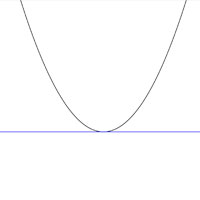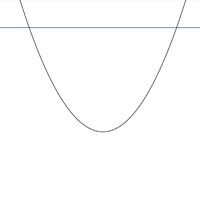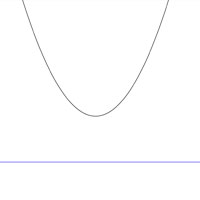SEARCH HOMEMath Central Quandaries & QueriesBarb, a student: I have a question I need help with please. What are the number of x and y intercepts that quadratic function can have and why? Thanks.Hi Barb,

Any quadratic function will have exactly one y-intercept. Parabolae (the shape of quadratic functions) infinity either increase or decrease so there has to be a y-value where x=0.

Think of the x-axis as a horizontal line, how many times could a horizontal line cross a parabola?A horizontal line will intersect a parabola 0, 1 or 2 times so that is the possible number of intercepts.

Hope this helps,

JaniceMath Central is supported by the University of Regina and The Pacific Institute for the Mathematical Sciences.